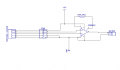#### sohamkul

Joined Jun 24, 2016
43
Hello Everyone,
I am working on a data acquisition project ,I have to get data from a pressure sensor of 5 pins
Pin1 = Vcc ,Pin2&3 = Sensor output , Pin4 = Shield , Pin5 = Ground
I want to power circuit using +5 volts.
Gain resistor is 390 ohm
so gain is roughly 130
Output of sensor is 2mV/V in this case 0 to 10mV(fullscale)
I have made a circuit on general purpose PCB which is shown below------Questions-------
2) Is the circuit Correct or i have messed up something ?
3)should reference voltage be grounded as i have done here ?

Last edited:

#### OBW0549

Joined Mar 2, 2015
3,566
2) Is the circuit Correct or i have messed up something ?
3)should reference voltage be grounded as i have done here ?
According to the AD620 data sheet, the voltage range for the Reference input (pin 5) is from +1.6V relative to the negative supply terminal to -1.6V relative to the positive supply terminal. In the case of 5 volt single supply operation as in your circuit, that would mean from +1.6V to +3.4V relative to ground. The data sheet doesn't specify what happens if that requirement is not met, but it is listed as a requirement nonetheless. I can't say for certain, but this may be the reason your circuit is misbehaving.

Related: If you look at Fig. 38 on p. 14 of the data sheet, "A Pressure Monitor Circuit that Operates on a 5 V Single Supply, " you can see that they've biased the Reference terminal at +2.00 volts. I suspect that's a clue as to why your circuit isn't working.

Last edited:
•sohamkul

#### sohamkul

Joined Jun 24, 2016
43
So if i just replicate the circuit i.e supply ref pin 2volt that would do ?
Can i use the voltage divider circuit a simple one to supply 2 volts ? instead of using AD705 will it be ok ?
Also if i power the circuit with -5volts to +5 volts ...will the same circuit work then ??
Thank you !

#### OBW0549

Joined Mar 2, 2015
3,566
So if i just replicate the circuit i.e supply ref pin 2volt that would do ?
Since that circuit appears as an example in the data sheet, I would assume so.

Can i use the voltage divider circuit a simple one to supply 2 volts ? instead of using AD705 will it be ok ?
No. As explained in the data sheet, pin 5 must be driven from a low impedance source, hence the AD705. Driving pin 5 from a resistive source (e.g., a voltage divider) will screw up both the amplifier gain and its common-mode rejection.

Also if i power the circuit with -5volts to +5 volts ...will the same circuit work then ??
Yes; if you provide a -5V supply for -Vs, then you would be free to simply ground the Reference input pin as you originally planned to do.

•sohamkul

#### sohamkul

Joined Jun 24, 2016
43
Thank you That was a very helpful explanation !!

#### OBW0549

Joined Mar 2, 2015
3,566
You're welcome. Glad I could help.

•sohamkul